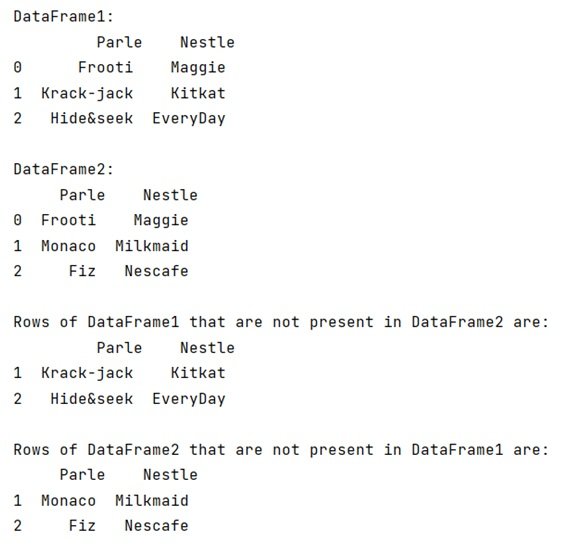# Pandas get rows which are NOT in other DataFrame

Given two DataFrames, we need to find the rows of a DataFrame which are not present in another DataFrame.
Submitted by Pranit Sharma, on May 07, 2022

## Getting rows which are NOT in other pandas DataFrame

For this purpose, we are going to merge two DataFrames, and then we will filter which row is present in another DataFrame and which is not.

Note

To work with pandas, we need to import pandas package first, below is the syntax:

```import pandas as pd
```

Let us understand with the help of an example:

## Python program to get rows which are NOT in other pandas DataFrame

```# Importing pandas package
import pandas as pd

# Defining two DataFrames
df1 = pd.DataFrame(data = {'Parle':['Frooti','Krack-jack','Hide&seek'],
'Nestle':['Maggie','Kitkat','EveryDay']
})

df2 = pd.DataFrame(data = {'Parle':['Frooti','Monaco','Fiz'],
'Nestle':['Maggie','Milkmaid','Nescafe']

})

# Display Separate DataFrames
print("DataFrame1:\n",df1,"\n")

print("DataFrame2:\n",df2,"\n")

# Merging two DataFrames on columns
merged = df1.merge(df2,on=['Parle','Nestle'])

# Filtering rows of df1
result = df1[(~df1.Parle.isin(merged.Parle))&(~df1.Nestle.isin(merged.Nestle))]

# Filtering rows of df2
result2 = df2[(~df2.Parle.isin(merged.Parle))&(~df2.Nestle.isin(merged.Nestle))]

# Display Result
print("Rows of DataFrame1 that are not present in DataFrame2 are:\n",result,"\n")

# Display Result2
print("Rows of DataFrame2 that are not present in DataFrame1 are:\n",result2)
```

### Output

The output of the above program is: